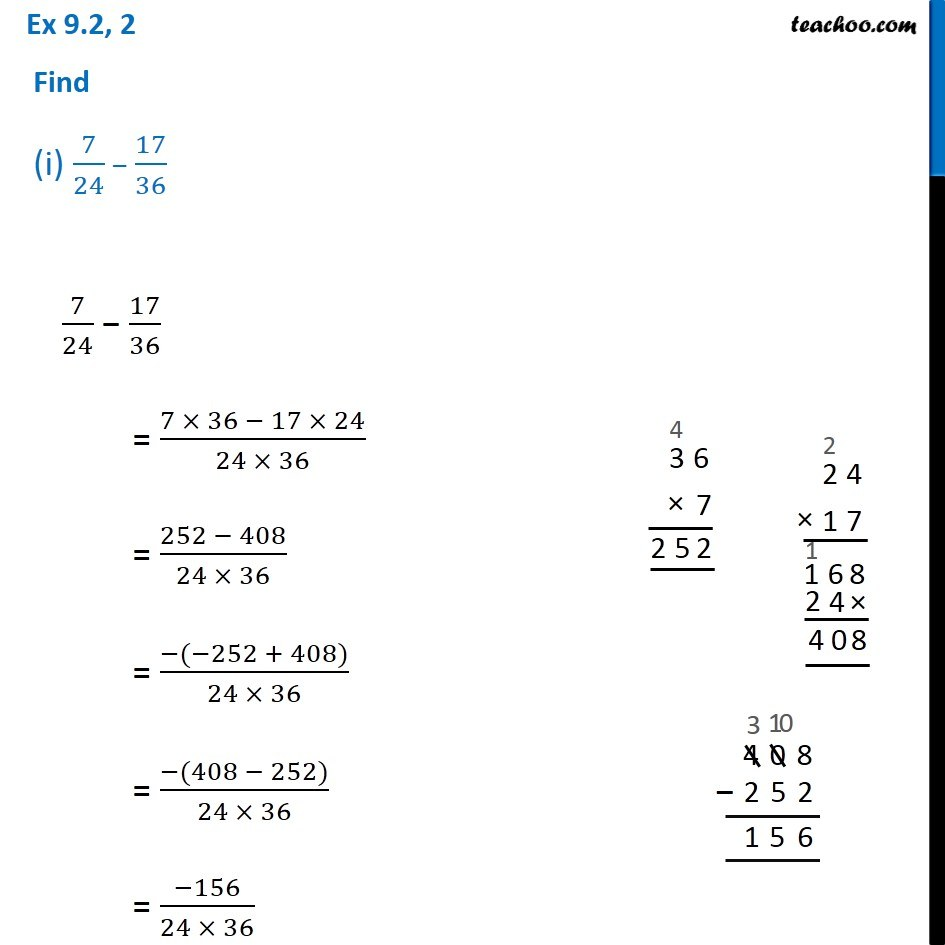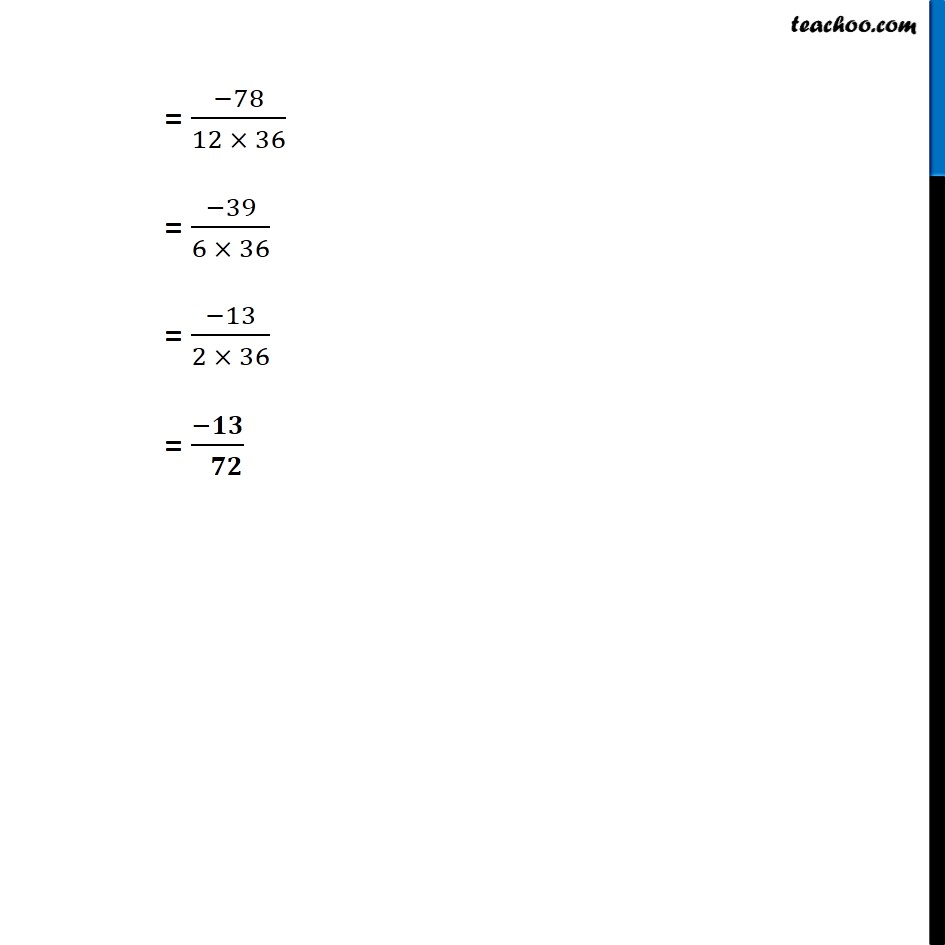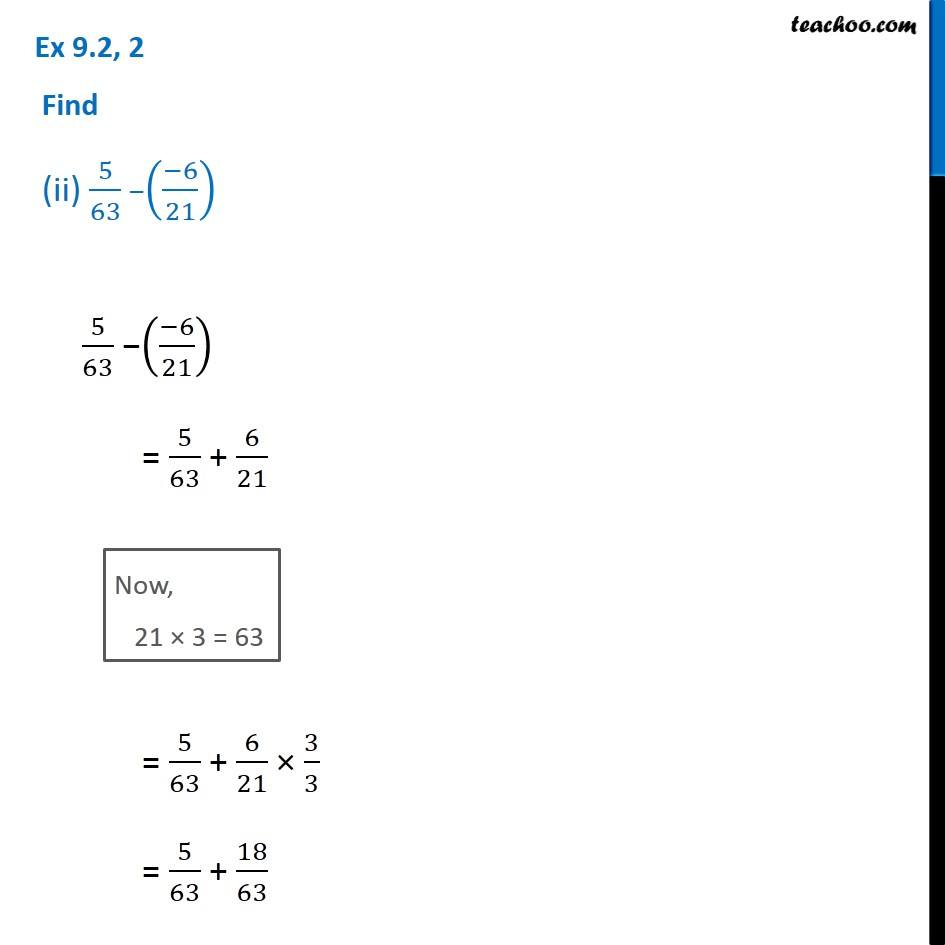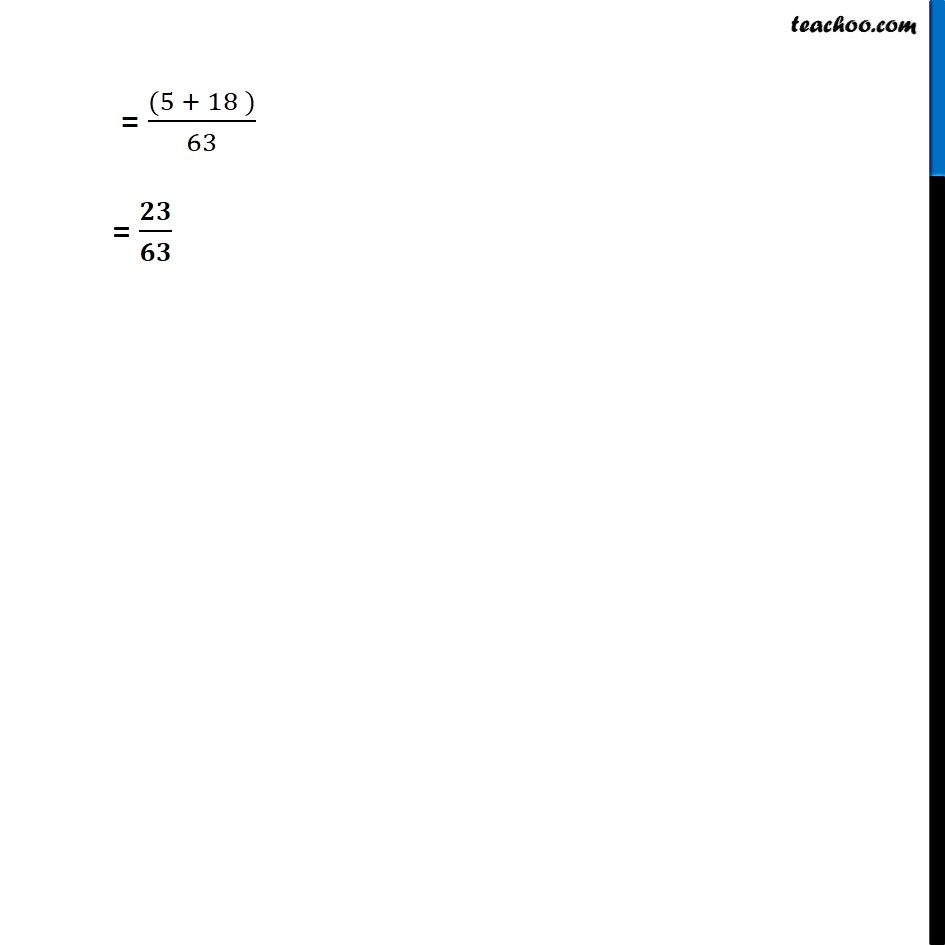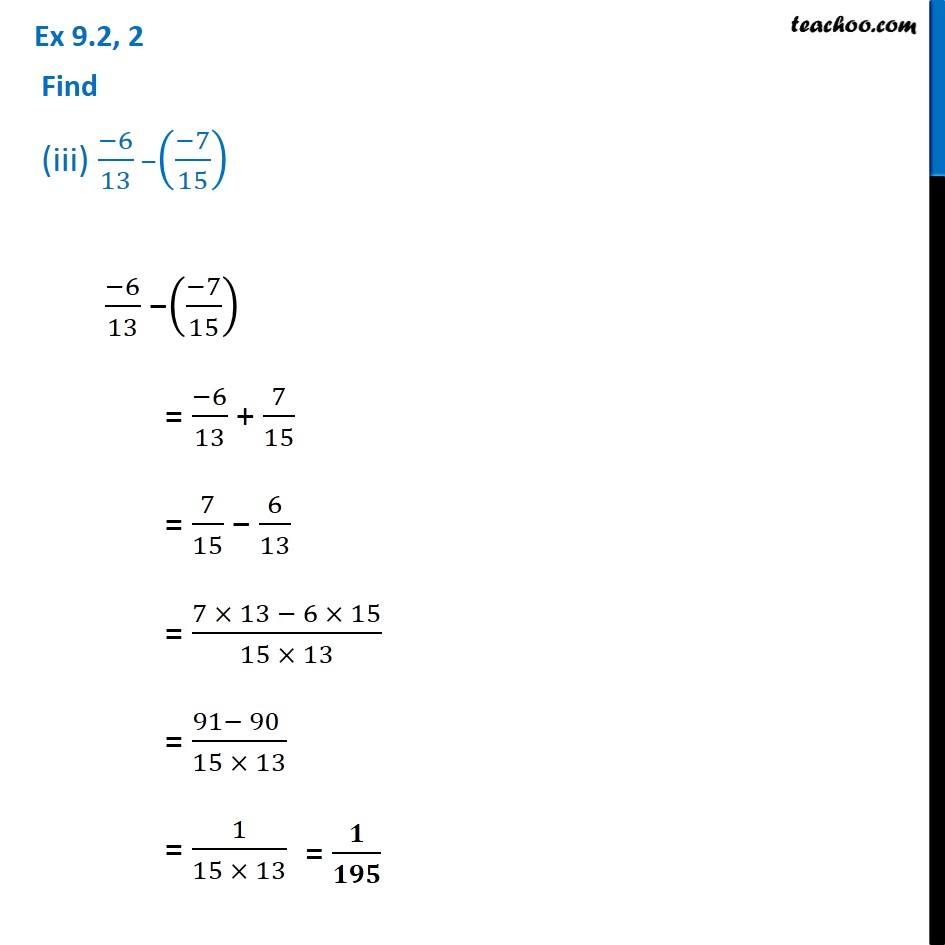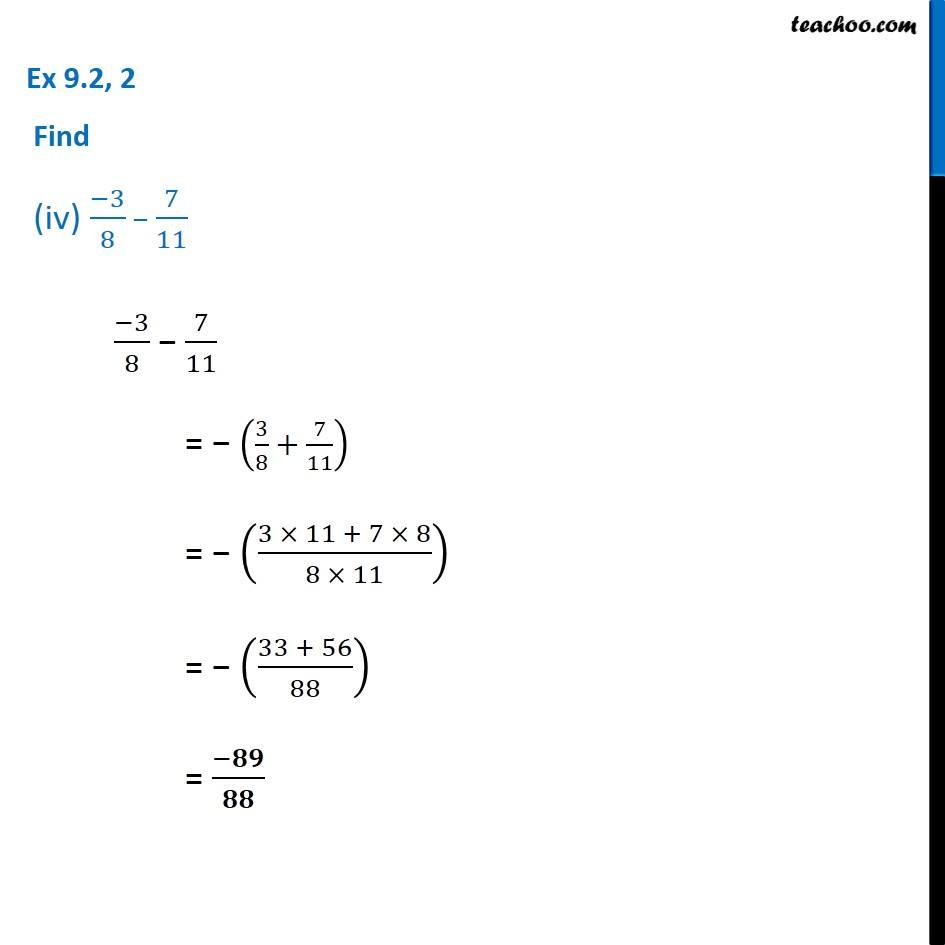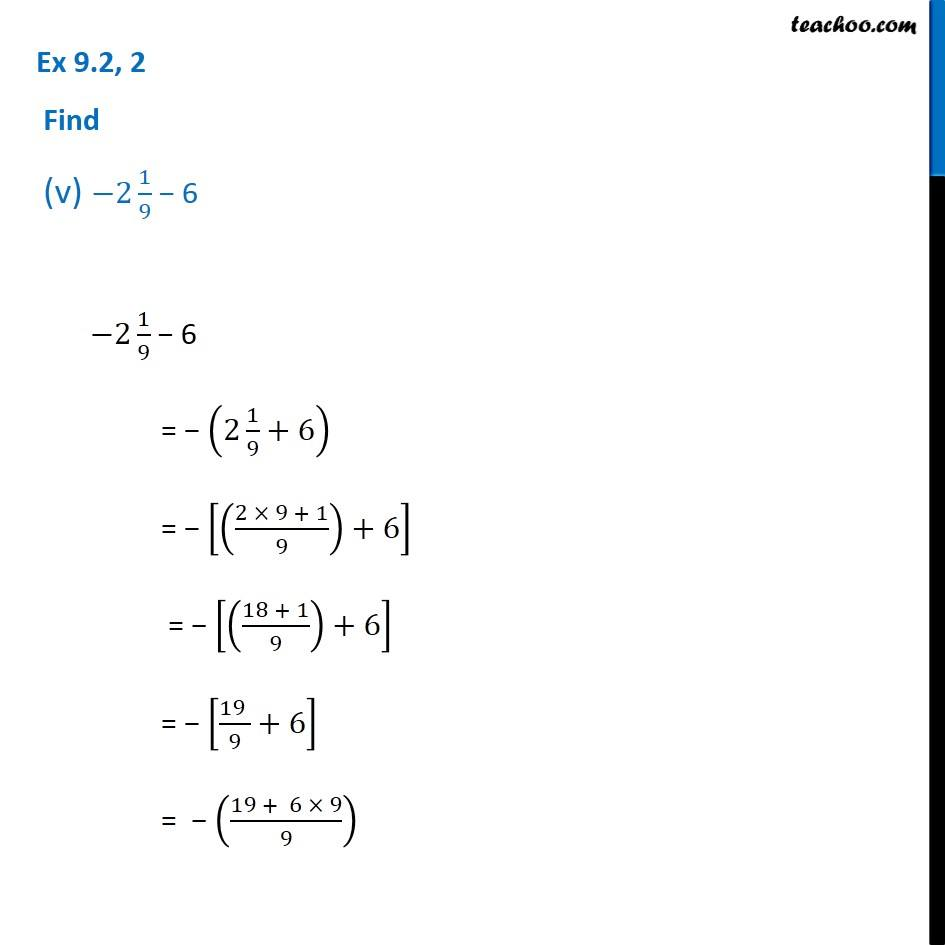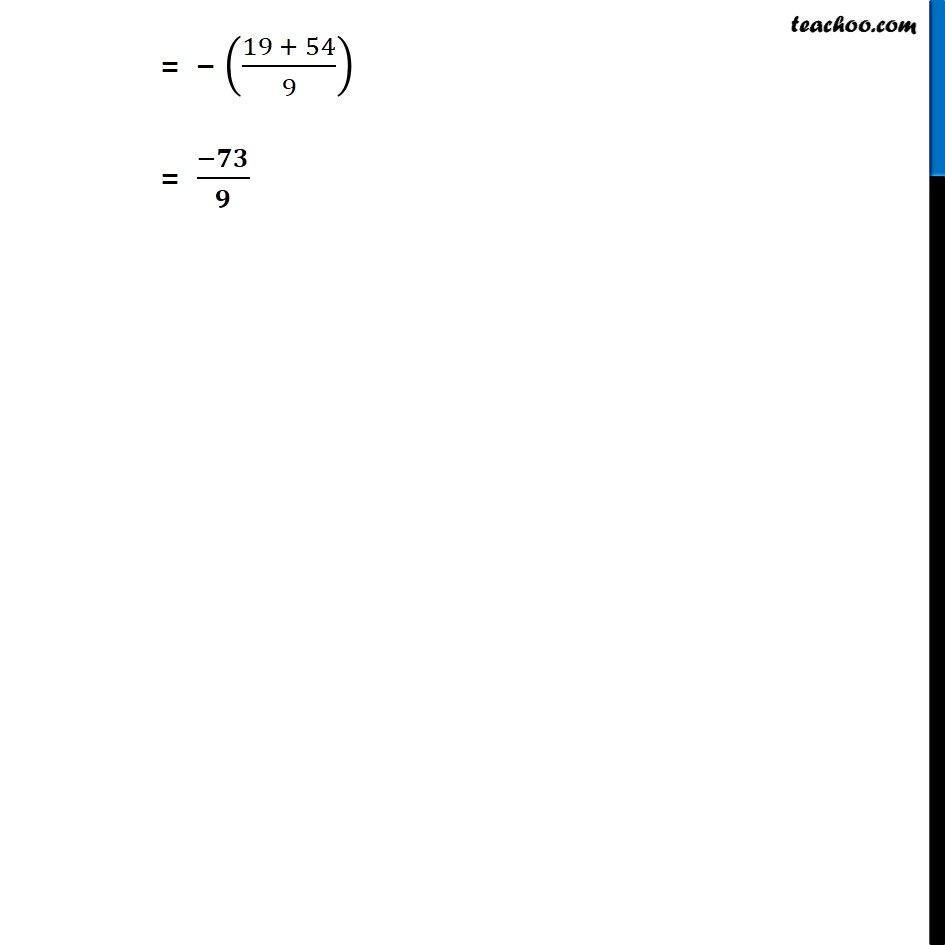1. Chapter 9 Class 7 Rational Numbers
2. Concept wise
3. Addition & Subtraction of rational numbers

Transcript

Ex 9.2, 2 Find (i) 7/24 − 17/36 7/24 − 17/36 = (7 × 36 − 17 × 24)/(24 × 36) = (252 − 408)/(24 × 36) = (−(−252 + 408))/(24 × 36) = (−(408 − 252))/(24 × 36) = (−156)/(24 × 36) = (−78)/(12 × 36) = (−39)/(6 × 36) = (−13)/(2 × 36) = (−𝟏𝟑)/( 𝟕𝟐) Ex 9.2, 2 Find (ii) 5/63 −((−6)/21) 5/63 −((−6)/21) = 5/63 + 6/21 Now, 21 × 3 = 63 = 5/63 + 6/21 × 3/3 = 5/63 + 18/63 = ((5 + 18 ))/63 = 𝟐𝟑/𝟔𝟑 Ex 9.2, 2 Find (iii) (−6)/13 −((−7)/15) (−6)/13 −((−7)/15) = (−6)/13 + 7/15 = 7/15 − 6/13 = (7 × 13 − 6 × 15)/(15 × 13) = (91− 90 )/(15 × 13) = 1/(15 × 13) = 𝟏/𝟏𝟗𝟓 Ex 9.2, 2 Find (iv) (−3)/8 − 7/11 (−3)/8 − 7/11 = − (3/8+7/11) = − ((3 × 11 + 7 × 8)/(8 × 11)) = − ((33 + 56)/88) = (−𝟖𝟗)/𝟖𝟖 Ex 9.2, 2 Find (v) −2 1/9 − 6 −2 1/9 − 6 = − (2 1/9+6) = − [((2 × 9 + 1)/9)+6] = − [((18 + 1)/9)+6] = − [(19 )/9+6] = − ((19 + 6 × 9)/9) = − ((19 + 54)/9) = (−𝟕𝟑)/𝟗

Addition & Subtraction of rational numbers